Examples

Chapter 11 Class 12 Three Dimensional Geometry
Serial order wise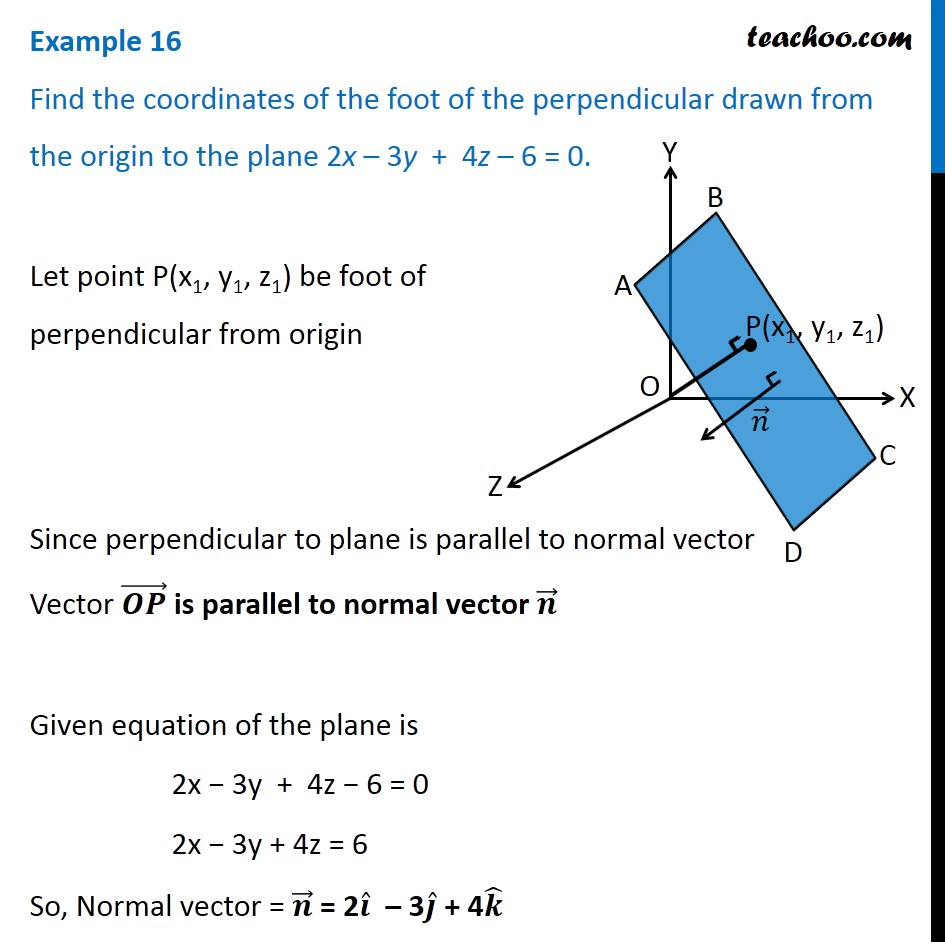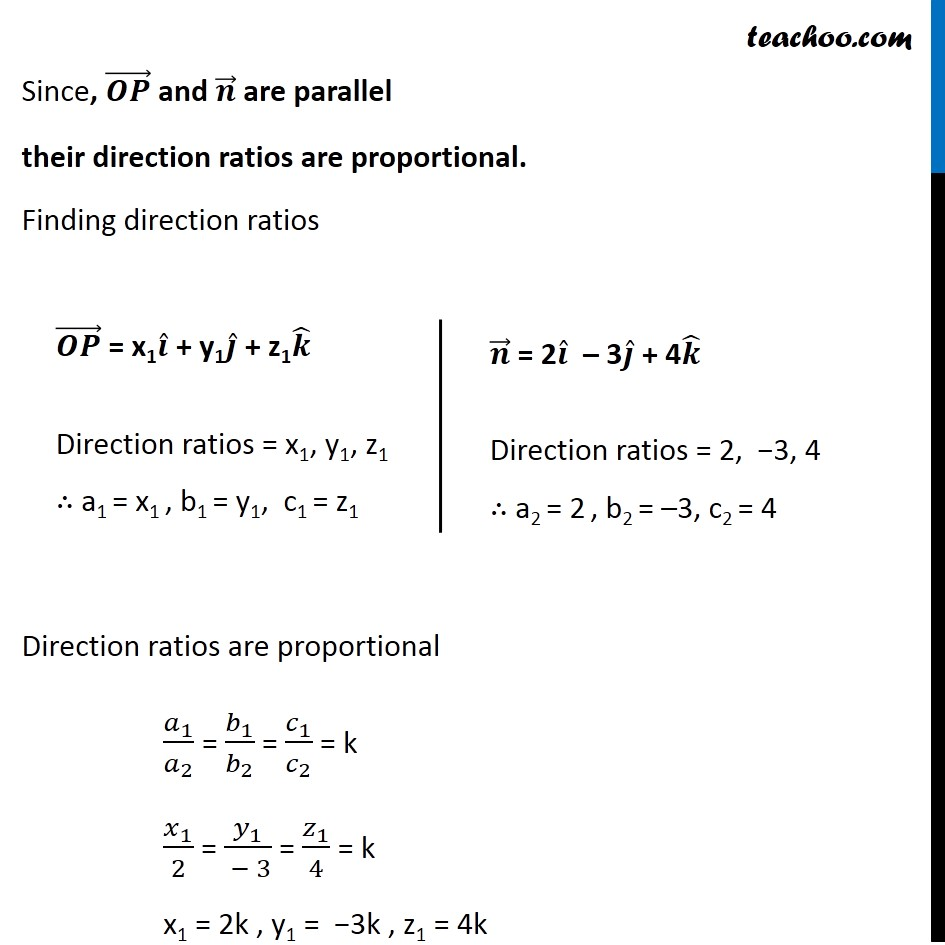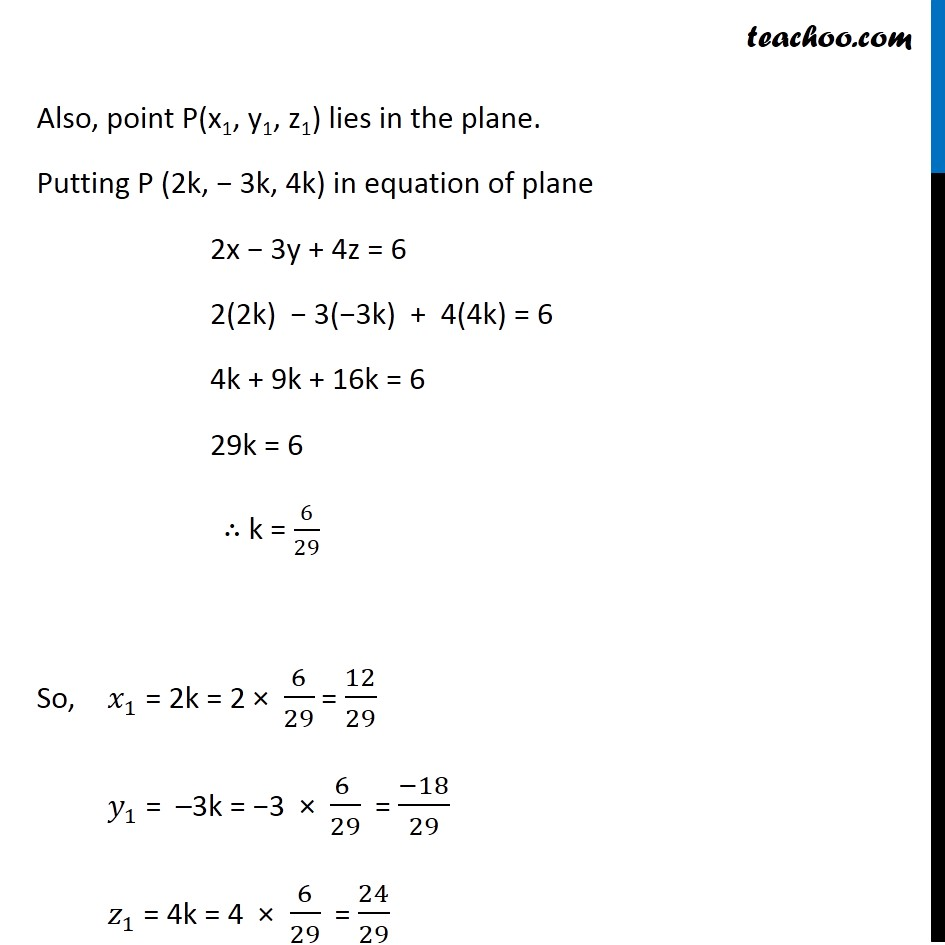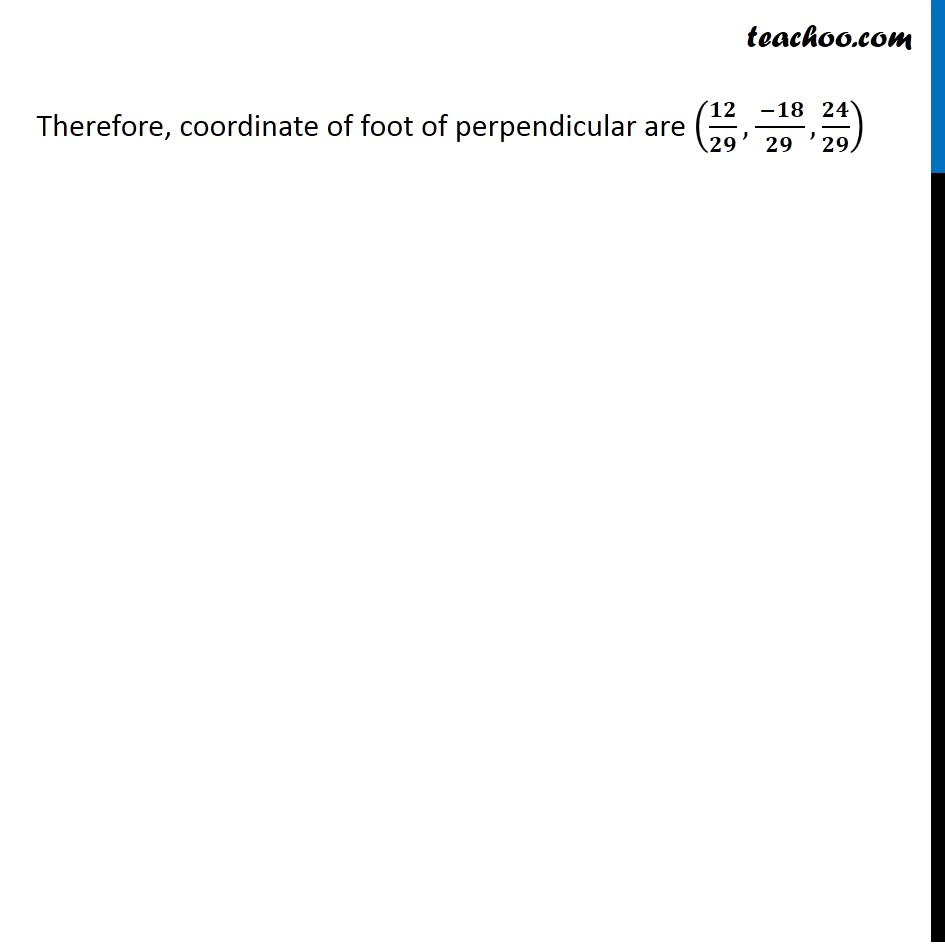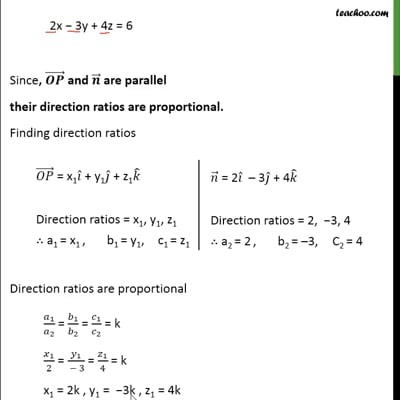This video is only available for Teachoo black users

Solve all your doubts with Teachoo Black (new monthly pack available now!)

### Transcript

Example 16 Find the coordinates of the foot of the perpendicular drawn from the origin to the plane 2x – 3y + 4z – 6 = 0. Let point P(x1, y1, z1) be foot of perpendicular from origin Since perpendicular to plane is parallel to normal vector Vector (𝑶𝑷) ⃗ is parallel to normal vector 𝒏 ⃗ Given equation of the plane is 2x − 3y + 4z − 6 = 0 2x − 3y + 4z = 6 So, Normal vector = 𝒏 ⃗ = 2𝒊 ̂ – 3𝒋 ̂ + 4𝒌 ̂ Since, (𝑶𝑷) ⃗ and 𝒏 ⃗ are parallel their direction ratios are proportional. Finding direction ratios Direction ratios are proportional 𝑎_1/𝑎_2 = 𝑏_1/𝑏_2 = 𝑐_1/𝑐_2 = k 𝑥_1/2 = 𝑦_1/( − 3) = 𝑧_1/4 = k x1 = 2k , y1 = −3k , z1 = 4k (𝑶𝑷) ⃗ = x1𝒊 ̂ + y1𝒋 ̂ + z1𝒌 ̂ Direction ratios = x1, y1, z1 ∴ a1 = x1 , b1 = y1, c1 = z1 𝒏 ⃗ = 2𝒊 ̂ – 3𝒋 ̂ + 4𝒌 ̂ Direction ratios = 2, −3, 4 ∴ a2 = 2 , b2 = –3, c2 = 4 Also, point P(x1, y1, z1) lies in the plane. Putting P (2k, − 3k, 4k) in equation of plane 2x − 3y + 4z = 6 2(2k) − 3(−3k) + 4(4k) = 6 4k + 9k + 16k = 6 29k = 6 ∴ k = 6/29 So, 𝑥_1 = 2k = 2 × 6/29 = 12/29 𝑦_1 = –3k = −3 × (6 )/29 = (−18)/29 𝑧_1 = 4k = 4 × 6/29 = 24/29 Therefore, coordinate of foot of perpendicular are (𝟏𝟐/𝟐𝟗, ( −𝟏𝟖)/𝟐𝟗,𝟐𝟒/𝟐𝟗)